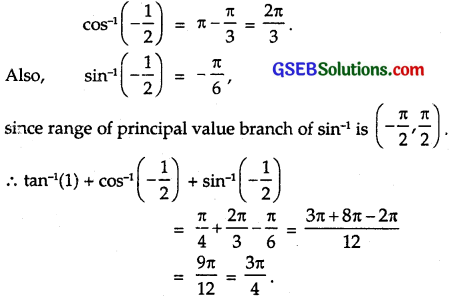# GSEB Solutions Class 12 Maths Chapter 2 Inverse Trigonometric Functions Ex 2.1

Gujarat Board GSEB Textbook Solutions Class 12 Maths Chapter 2 Inverse Trigonometric Functions Ex 2.1 Textbook Questions and Answers.

## Gujarat Board Textbook Solutions Class 12 Maths Chapter 2 Inverse Trigonometric Functions Ex 2.1Find the principal values of the following:

Question 1.
sin-1(-$$\frac { 1 }{ 2 }$$)
Solution:
Let sin-1(-$$\frac { 1 }{ 2 }$$) = y
∴ sin y = $$\frac { 1 }{ 2 }$$ = – sin$$\frac { π }{ 6 }$$ = sin(-$$\frac { π }{ 6 }$$)
The range of principal value branch of sin-1 is [-$$\frac { π }{ 2 }$$, $$\frac { π }{ 2 }$$].
Hence, principal value of sin-1(-$$\frac { 1 }{ 2 }$$) is – $$\frac { π }{ 6 }$$.

Question 2.
cos-1$$\left(-\frac{\sqrt{3}}{2}\right)$$
Solution:
Let cos-1$$\left(\frac{\sqrt{3}}{2}\right)$$ = y
∴ cos y = $$\left(\frac{\sqrt{3}}{2}\right)$$ = – cos$$\frac { π }{ 6 }$$
The range of principal value branch is (0, π)
∴ principal value of cos-1$$\left(\frac{\sqrt{3}}{2}\right)$$ is $$\frac { π }{ 6 }$$.Question 3.
cosec-1(2)
Solution:
Let cosec-1(2) = y
∴ cosec y = 2 = cosec$$\frac { π }{ 6 }$$
The range of principal value branch of cosec-1 is [-$$\frac { π }{ 2 }$$, $$\frac { π }{ 2 }$$] – 
∴ principal value of cosec-1(2) is $$\frac { π }{ 6 }$$.

Question 4.
tan-1(-$$\sqrt{3}$$)
Solution:
Let tan-1(-$$\sqrt{3}$$) = y
∴ tan y = 2 = – $$\sqrt{3}$$ = – tan$$\frac { π }{ 3 }$$ = tan(-$$\frac { π }{ 3 }$$)
The range of principal value branch of tan-1 is [-$$\frac { π }{ 2 }$$, $$\frac { π }{ 2 }$$]
∴ principal value of
tan-1(-$$\sqrt{3}$$) is – $$\frac { π }{ 3 }$$.

Question 5.
cos-1(-$$\frac { 1 }{ 2 }$$)
Solution:
Let cos-1(-$$\frac { 1 }{ 2 }$$) = y
∴ cos y = –$$\frac { 1 }{ 2 }$$ = – cos$$\frac { π }{ 3 }$$ = cos(π – $$\frac { π }{ 3 }$$
= cos $$\frac { 2π }{ 3 }$$
The range of principal value branch is (0, π)
∴ principal value of cos-1(-$$\frac { 1 }{ 2 }$$) is $$\frac { 2π }{ 3 }$$.Question 6.
tan-1(-1)
Solution:
Let tan-1(-1) = y.
∴ tan y = – 1 = tan$$\frac { π }{ 4 }$$) = tan($$\frac { -π }{ 4 }$$)
The range of principal value branch of tan-1 is [-$$\frac { π }{ 2 }$$, $$\frac { π }{ 2 }$$]
∴ principal value of tan-1(-1) is $$\frac { π }{4 }$$.

Question 7.
sec-1$$\left(-\frac{2}{\sqrt{3}}\right)$$
Solution:
Let sec-1$$\left(\frac{2}{\sqrt{3}}\right)$$ = y
∴ sec y = $$\left(\frac{2}{\sqrt{3}}\right)$$ = sec($$\frac { π }{ 6 }$$)
The range of principal value branch of sec-1 is [0, π], ($$\frac { π }{ 2 }$$)
∴ principal value of sec-1$$\left(\frac{2}{\sqrt{3}}\right)$$ is $$\frac { π }{6 }$$.

Question 8.
cot-1($$\sqrt{3}$$)
Solution:
Let cot-1($$\sqrt{3}$$) = y
∴ cot y = $$\sqrt{3}$$ = cot$$\frac { π }{ 6 }$$
The range of principal value branch of cot-1 is [0, π]
∴ principal value of cot-1$$\sqrt{3}$$ is $$\frac { π }{ 6 }$$.

Question 9.
cos-1$$\left(-\frac{1}{\sqrt{2}}\right)$$
Solution:
Let cos-1$$\left(-\frac{1}{\sqrt{2}}\right)$$ = y
∴ cos y = $$\left(-\frac{1}{\sqrt{2}}\right)$$ = cos($$\frac { π }{ 4 }$$) = cos(π – $$\frac { π }{ 4 }$$)
The range of principal value branch of cos-1 is [0, π].
∴ principal value of cos-1$$\left(-\frac{1}{\sqrt{2}}\right)$$ is $$\frac { 3π }{4 }$$.Question 10.
cosec-1 (-$$\sqrt{2}$$)
Solution:
Let cosec-1(-$$\sqrt{2}$$) = y
∴ cosec y = –$$\sqrt{2}$$ = – cosec$$\frac { π }{ 4 }$$ = cosec (- $$\frac { π }{ 4 }$$).
The range of principal value branch of cosec-1 is (- $$\frac { π }{ 2 }$$, $$\frac { π }{ 2 }$$) – {0}
∴ principal value of cosec-1(-$$\sqrt{2}$$) is – $$\frac { π }{ 4 }$$.

Question 11.
tan-1(1) + cos-1(-$$\frac { 1 }{ 2 }$$) + sin-1($$\frac { -1 }{ 2 }$$)
Solution:
tan-1(1) + cos-1(-$$\frac { 1 }{ 2 }$$) + sin-1($$\frac { -1 }{ 2 }$$)
Now, tan-1 1 = $$\frac { π }{ 4 }$$,
Since range of principal value branch of cos-1 is [0, π]. So,Question 12.
cos-1($$\frac { 1 }{ 2 }$$) + 2 sin-1($$\frac { 1 }{ 2 }$$)
Solution:
cos-1($$\frac { 1 }{ 2 }$$) + 2 sin-1($$\frac { 1 }{ 2 }$$)
Now, cos-1($$\frac { 1 }{ 2 }$$) = $$\frac { π }{ 3 }$$
and sin-1($$\frac { 1 }{ 2 }$$) = $$\frac { π }{ 6 }$$
∴ cos-1($$\frac { 1 }{ 2 }$$) + 2 sin-1($$\frac { 1 }{ 2 }$$)
= $$\frac { π }{ 3 }$$ + 2 x $$\frac { π }{ 6 }$$ = $$\frac { 2π }{ 3 }$$.

Question 13.
If sin-1 = y, then
(A) 0 ≤ y ≤ π
(B) – $$\frac { π }{ 2 }$$ ≤ y ≤ $$\frac { π }{ 2 }$$
(C) 0 < y < π
(D) – $$\frac { π }{ 2 }$$ < y $$\frac { π }{ 2 }$$
Solution:
The range of principal value branch of sin-1 is [-$$\frac { π }{ 2 }$$, $$\frac { π }{ 2 }$$]
∴ if sin-1 x = y, then – $$\frac { π }{ 2 }$$ ≤ y ≤ $$\frac { π }{ 2 }$$
∴ Part (B) is correct.Question 14.
tan-1$$\sqrt{3}$$ – sec-1(-2) is equal to
(A) π
(B) – $$\frac { π }{ 3 }$$
(C) $$\frac { π }{ 3 }$$
(D) $$\frac { 2π }{ 3 }$$
Solution:
tan-1$$\sqrt{3}$$ = $$\frac { π }{ 3 }$$
and sec-1(-2) = π – $$\frac { π }{ 3 }$$ = $$\frac { 2π }{ 3 }$$,
since principal value branch of sec-1 is [0, π] – {$$\frac { π }{ 2 }$$}
∴ tan-1$$\sqrt{3}$$ – sec-1(-2) = $$\frac { π }{ 3 }$$ – $$\frac { 2π }{ 3 }$$
= – $$\frac { π }{ 3 }$$
∴ Part (B) is correct.